# Dead Short: What is it? (vs Short Circuit vs Bolted Fault vs Ground Fault)

Contents

## What is a Dead Short?

A dead short is an electrical circuit that results in current flowing along an unintended path with no resistance or impedance. This results in an excessive current flowing through the circuit, which can damage equipment or cause electrical shocks to those nearby.

A dead short is difficult to track and diagnose as the current builds rapidly and trips the breaker immediately.

It is mainly caused due to direct connection between positive and negative power wires or a direct connection between the positive wire and the ground.

Dead shorts are very dangerous because it causes a high amount of current to flow through the circuit.

## Dead Short vs Short Circuit

To understand the difference between a dead short and a short circuit, let’s take an example. Consider a voltage difference between two points is 150 V.

If we measure the voltage between two points in normal conditions, it shows 150 V. But, if the voltage between two points is less than 150 V, it is called a short circuit.

Some voltage drop occurs during the short circuit, and some resistance appears between these two points.

If the measured voltage is 0 V, it is called dead short. It means there is zero resistance of a circuit.

The difference between the normal condition, short-circuit, and dead-short describes in the figure below.

## Dead Short vs Bolted Fault

A bolted fault is defined as a fault with zero impedance. It produces extreme fault current in the system.

When all conductors are connected to the ground with a metallic conductor, the fault is known as a bolted fault.

The bolted fault (bolted short) is quite similar to the dead short. As in the dead short also, the resistance is zero.

## Dead Short vs Ground Fault

The ground fault occurs in the power system when the hot wire (live wire) accidentally connected with the earth wire or grounded equipment frame.

In this condition, the frame of equipment energies with dangerous voltage. In the ground fault, there is some amount of ground resistance present. And the fault current depends on the ground resistance.

Therefore, the ground fault is different from the dead short.

## Example of a Dead Short

To understand the dead short, let’s take an example. Consider a network having three resistors connected in series, as shown in the figure below.

In normal conditions, the current passes through the circuit are I ampere. And the total resistance of a circuit is REQ.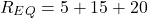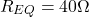According to ohm’s law;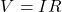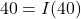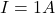Therefore, the current that passes through the circuit is 1 A in normal condition.

If we short battery terminals by a metallic wire or very low resistance (ideally zero resistance), the circuit looks like the figure below.

As points A and B are shorted with ideally zero resistance, it is known as dead short. And the current that passes through the resisters is zero.

All the current will flow through the shorted terminals. Because the current always follows a low resistance path.

Due to zero resistance, the current passes through terminals A and B is;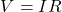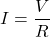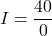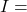From the calculation, infinite current will flow through the point A and B. But practically, there is some amount of current that will flow. And this current is very high compared to the standard current (1 A).

In a power system network, you can consider a part of a network instead of three resisters. The circuit will look like the figure below.

Want To Learn Faster? 🎓
Get electrical articles delivered to your inbox every week.
No credit card required—it’s 100% free.